## Saturday, 9 August 2014

### chapter 1 Lines and Angles

The following pages briefly summarize some of the important results from plane geometry that we’ll encounter frequently in pre-college mathematics. No proofs have been provided for these results – you are expected to work out the proofs on your own.
Theorem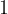$1$.
If a triangle is isosceles (i.e. it has two equal sides) then the angles opposite the equal sides are equal. Also, if two angles of a triangle are equal then the two sides opposite the equal angles are equal, so that the triangle is isosceles.Lines and angles
In the following diagram the two horizontal lines are parallel. The line cutting the two parallel lines is called a transversal. Angles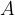$A$ and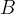$B$ are called alternate angles,$A$ and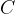$C$ are corresponding angles, and angles$A$ and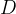$D$ are supplementary angles. Alternate angles and corresponding angles are equal, and pairs of supplementary angles sum to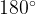${180^ \circ }$.Theorem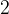$2$
The sum of the interior angles of a triangle is${180^ \circ }$.Theorem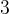$3$.
An exterior angle of a triangle equals the sum of the two non-adjacent interior angles.Theorem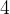$4$
The sum of the interior angles of an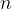$n$-sided polygon is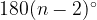$180{(n - 2)^ \circ }$.